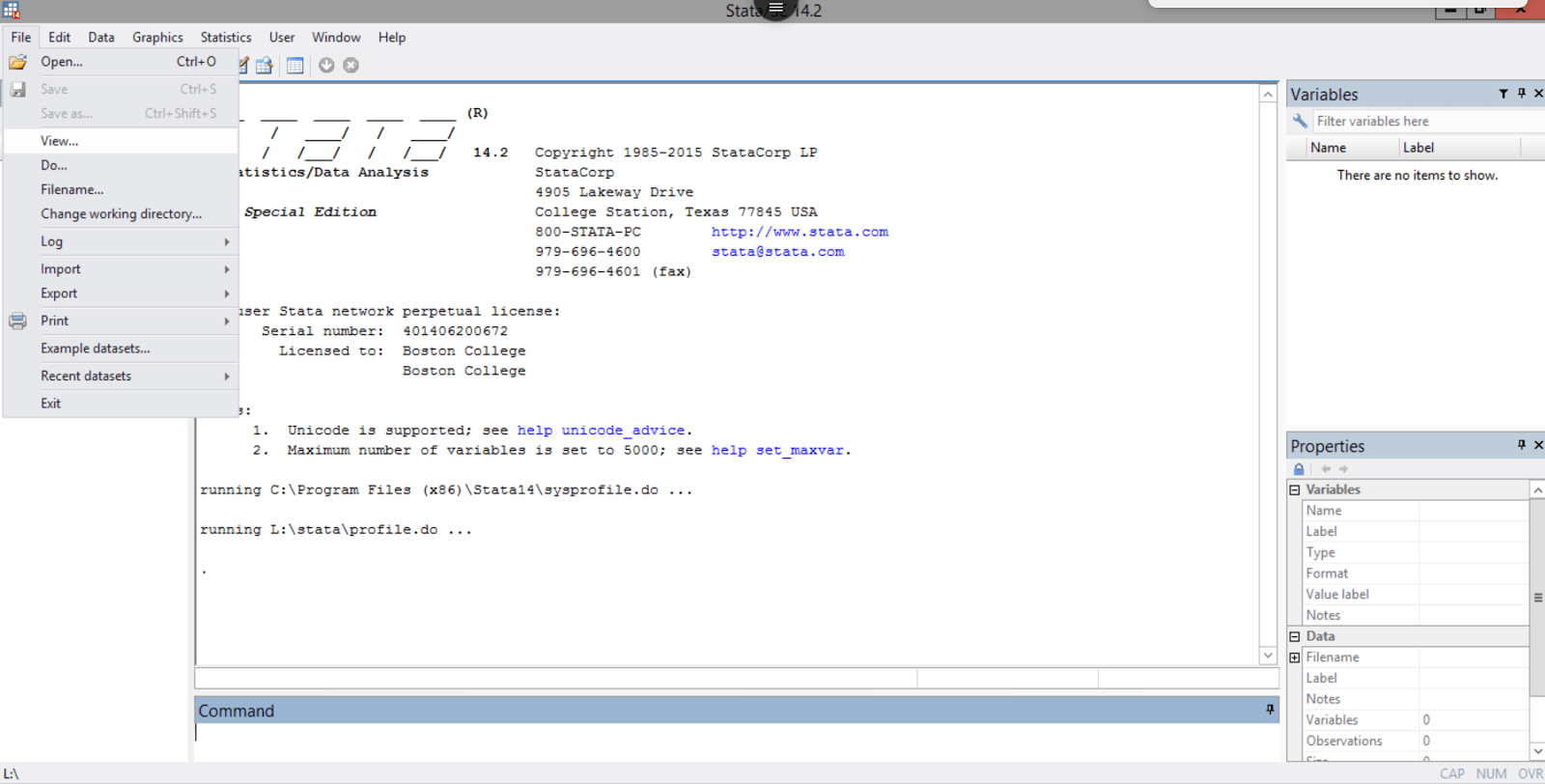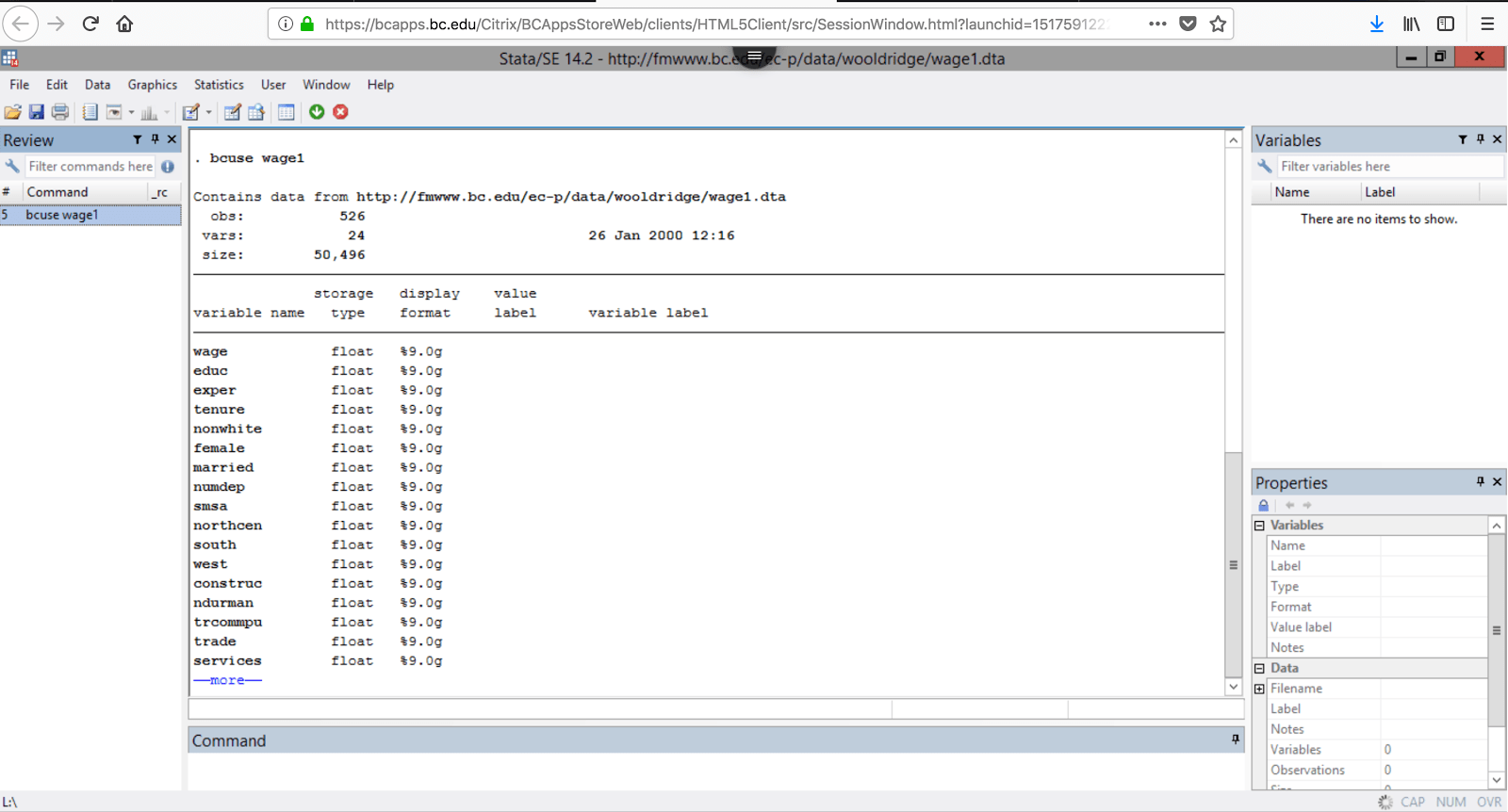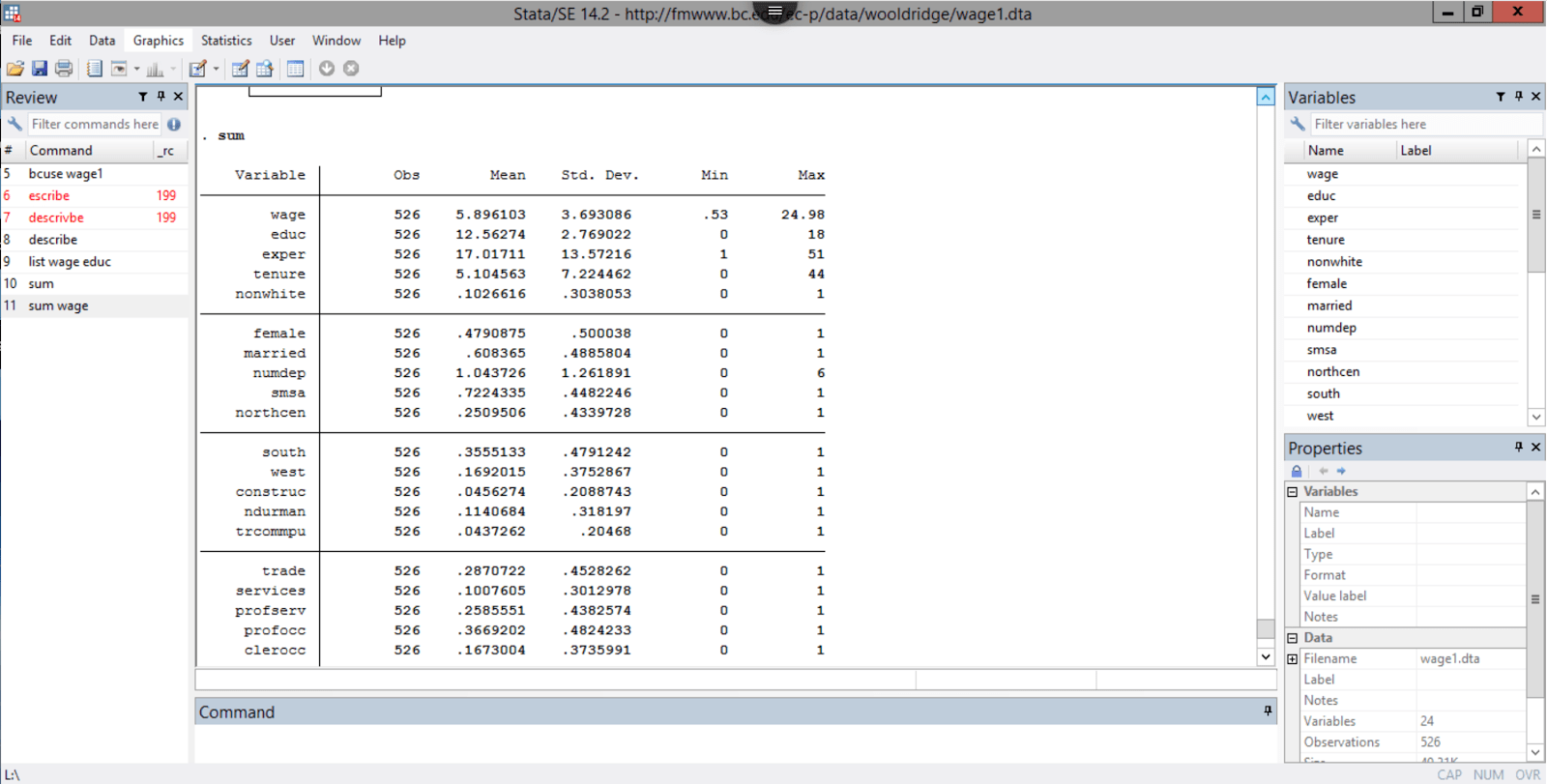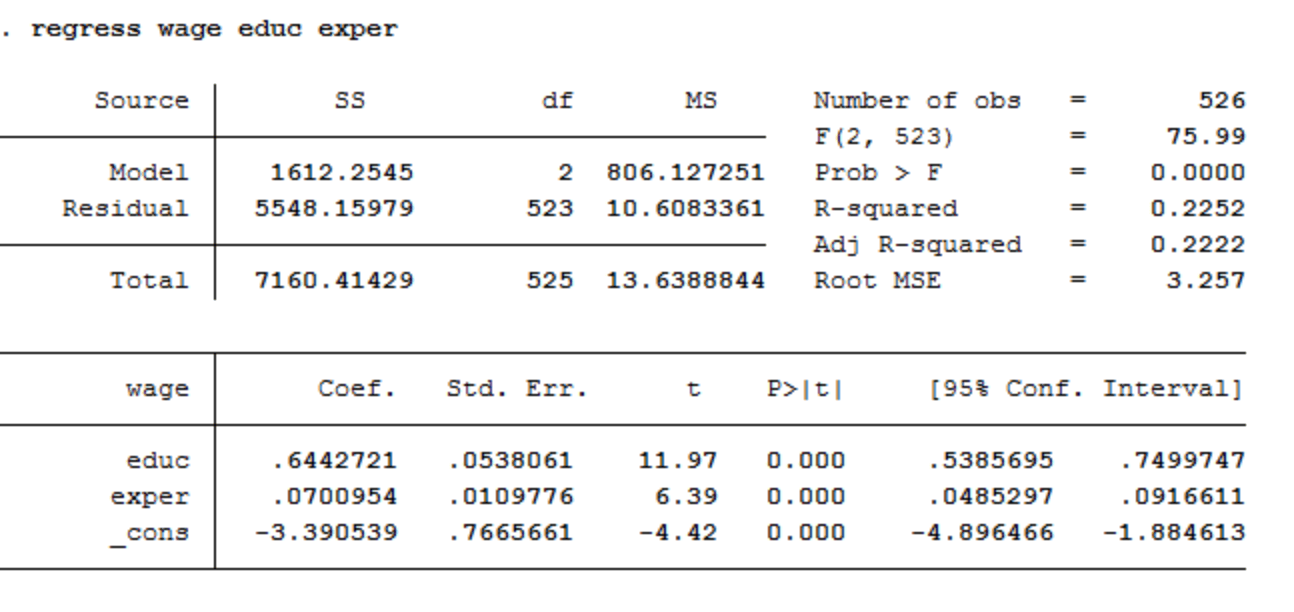# Intro to Stata

This tutorial was written by Digital Studio Student Assistant, Michael Scarpetti. Learn more about Michael and our other student assistants here.

In this tutorial, the goal is to gain a basic understanding of Stata, a statistical analysis program that is used across many disciplines. While the interface of Stata may seem daunting, once you know how to use the functions available to you, using it becomes a breeze. Today, we will go over the basics of Stata, such as loading and importing different types of files, basic data analysis tools, and basic regression functions. While this program is used by many disciplines, the main goal of today is to show how it can be used to perform statistical analysis.

The first step is to load the data into the program. You will need a dataset, which can be in the form of a .dta, .xls, .csv, and other text files. Seen below is the menu needed to access the file loading. If it is a .dta file, you can click open and find it in your computer. If it is any other file, you will have to go down to import and do the same.### Basic Data Inquiries

Listed Below are 4 essential statistical inquiries you can make and what they do. In order to do these functions, simply type the wording into the command section at the bottom.

• DESCRIBE: tells you about the dataset. Includes the number of observations, variables, and the size of the file. It also lists the various variables.• LIST (VAR 1 VAR 2): this command allows you to list out each observation of any variables you desire. In this case, you need to type the specific variable after the word LIST to get the desired outcome.
• SUMMARIZE: this command gives you the basic statistical numbers that you usually need to see when doing statistical analysis. It tells you the number of observations per variable, each variable’s mean, each standard deviation, and each variable’s maximum and minimum. The command can be shortened to SUM, and you can also summarize specific variables instead of the whole dataset if you wish.• CORRELATE (VAR1, VAR2, etc.): the correlate command gives you a correlation table of all variables or specific variables if desired. The command can also be shortened to CORR, as seen below.### Running Regression on Stata

One key feature of Stata is its ability to run regressions on a number of different variables. Regression helps us understand how multiple variables relate to each other. This is a vital command when doing statistical work. The command for running a regression is: REGRESS (VAR1, VAR2, VAR3)As seen above, the regression analysis gives all of the important statistical numbers to help you understand a set of numbers. The command gives the coefficient, the standard error, the 95% confidence interval, and the R-Squared number. Doing all of this by hand would be tiresome and tedious work, so Stata saves a lot of time when finding doing statistical analysis.

### Conclusion

I hope this tutorial helps you in your pursuit of learning Stata. The Program is a great tool to use when analyzing datasets and large amounts of observations. If there is something you are unsure of and want more clarity on something, check out the Stata handbook, which is written by the creators of Stata. The link is listed below. I hope you learned something and good luck with your statistical endeavors!